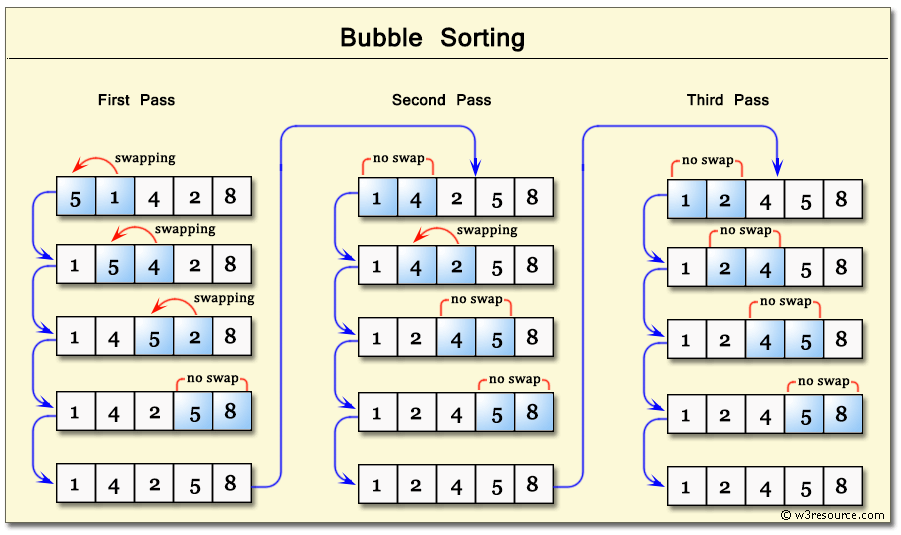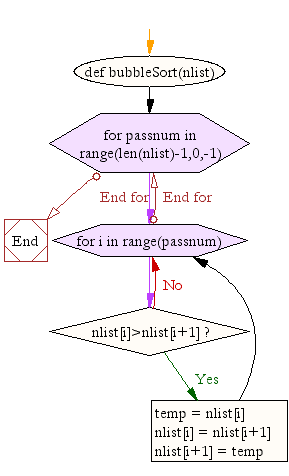﻿ Python: Bubble sort - w3resource# Python: Bubble sort

## Python Search and Sorting : Exercise-4 with Solution

Write a Python program to sort a list of elements using the bubble sort algorithm.
Note : According to Wikipedia "Bubble sort, sometimes referred to as sinking sort, is a simple sorting algorithm that repeatedly steps through the list to be sorted, compares each pair of adjacent items and swaps them if they are in the wrong order. The pass through the list is repeated until no swaps are needed, which indicates that the list is sorted. The algorithm, which is a comparison sort, is named for the way smaller elements "bubble" to the top of the list. Although the algorithm is simple, it is too slow and impractical for most problems even when compared to insertion sort. It can be practical if the input is usually in sort order but may occasionally have some out-of-order elements nearly in position.

Step by step pictorial presentation :Sample Solution:-

Python Code:

``````def bubbleSort(nlist):
for passnum in range(len(nlist)-1,0,-1):
for i in range(passnum):
if nlist[i]>nlist[i+1]:
temp = nlist[i]
nlist[i] = nlist[i+1]
nlist[i+1] = temp

nlist = [14,46,43,27,57,41,45,21,70]
bubbleSort(nlist)
print(nlist)
```
```

Sample Output:

```[14, 21, 27, 41, 43, 45, 46, 57, 70]
```

Flowchart:Python Code Editor :

What is the difficulty level of this exercise?

Test your Programming skills with w3resource's quiz.

﻿

## Python: Tips of the Day

The Zip() Function:

```>>> students = ('John', 'Mary', 'Mike')
>>> ages = (15, 17, 16)
>>> scores = (90, 88, 82, 17, 14)
>>> for student, age, score in zip(students, ages, scores):
...     print(f'{student}, age: {age}, score: {score}')
...
John, age: 15, score: 90
Mary, age: 17, score: 88
Mike, age: 16, score: 82
>>> zipped = zip(students, ages, scores)
>>> a, b, c = zip(*zipped)
>>> print(b)
(15, 17, 16)
```